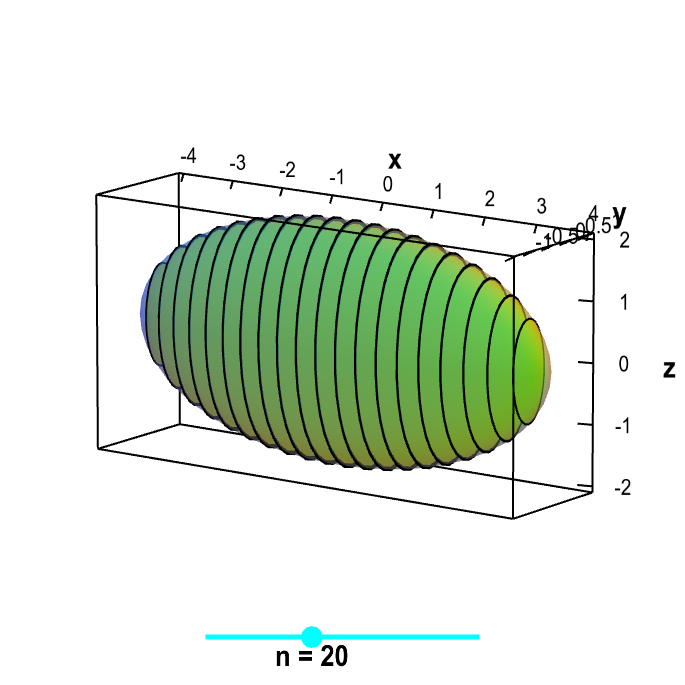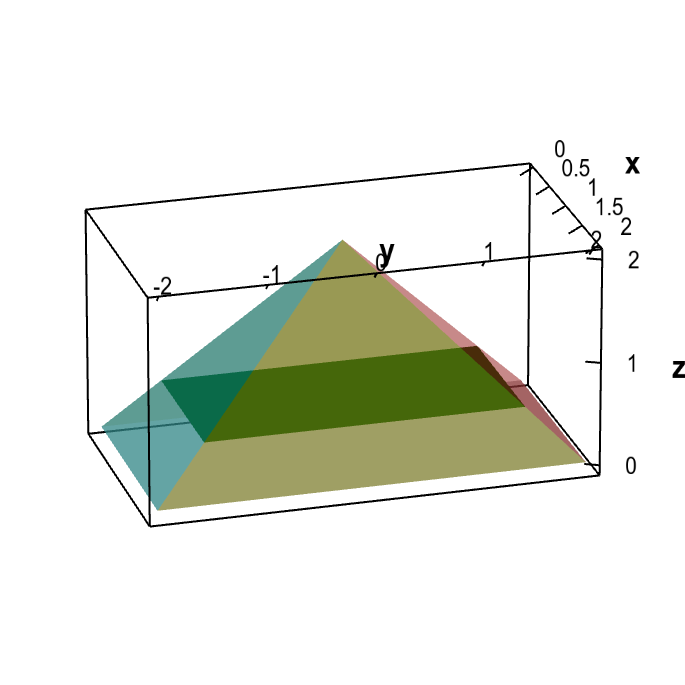# Math Insight

### The cross section method for determining triple integral bounds

One tricky part of calculating define triple integrals is determining the bounds of integration. To help you in this endeavor, we outline a couple methods for reducing the task of finding these bounds to the simpler task of finding bounds on a double integral combined with a single integral. One such method is one we call the “shadow method,” which we describe another page. Here we describe a method we call the “cross section method.”

To begin, imagine taking a big meat cleaver and chopping the three-dimensional region $\dlv$ into slices perpendicular to one of the coordinate axes (in a similar manner to the way in which we take cross sections of a surface). If you view the axis perpendicular to the slices as being vertical, then you could view the region $\dlv$ as being composed of a bunch of these cross sections stacked on top of each other. For example, for the below ellipsoidal region, we would view the $x$-axis as being vertical.Stack of cross sections. Inside the transparent ellipsoidal region $x^2/16+y^2+z^2/4 \le 1$ are a stack of cross sections that are cut perpendicular to the $x$-axis. If you view the $x$-axis as being vertical, then the cross sections are stacked on top of each other. You can drag the cyan point on the slider to change the number of cross sections.

Unless $\dlv$ happens to be a cylinder, the size and/or shape of the cross section will vary as a function of the location along the “vertical” coordinate axis (i.e., the coordinate axis perpendicular to the cross sections). The integral over $\dlv$ will correspond to the double integrals over all these cross sections, summed up from the bottom cross section up to the top cross section. If the “vertical” axis is the $z$-axis, we can write the integral of $f(x,y,z)$ over $\dlv$ as $$\iiint_{\dlv} f(x,y,z)dV = \int_{\text{bottom}}^{\text{top}} \left(\iint_{\text{cross section}(z)} f(x,y,z) dx\,dy\right)dz.$$ On the other hand, if the $y$-axis is the “vertical” axis, the integral is $$\iiint_{\dlv} f(x,y,z)dV = \int_{\text{bottom}}^{\text{top}} \left(\iint_{\text{cross section}(y)} f(x,y,z) dx\,dz\right)dy.$$ If the $x$-axis is the “vertical” axis, the integral is $$\iiint_{\dlv} f(x,y,z)dV = \int_{\text{bottom}}^{\text{top}} \left(\iint_{\text{cross section}(x)} f(x,y,z) dy\,dz\right)dx.$$

Note that the differences between the cross section method and the shadow method. For the shadow method, the double integral is on the outside (with constant bounds) and the single integral is on the inside (with variable bounds). In contrast, for the cross section method, the double integral is on the inside (with variable bounds) and the single integral is on the outside (with constant bounds). This result is consistent with the idea that the cross sections change size or shape with height while the shadow is just a single shape.

We illustrate the cross section method with an example.

#### Example

Let $\dlv$ be the pyramid bounded by the planes $z=0$, $z=4-2x$, $z=2-y$, $z=2x$, and $z=2+y$. Let the density of the pyramid at point $(x,y,z)$ be $f(x,y,z)=xz$. Find the mass of the pyramid.

Solution: The mass of the pyramid is the integral of its density: $$\text{mass of pyramid} = \iiint_{\dlv} f(x,y,z) dV,$$ where $\dlv$ is the pyramid. The first task is to determine the integration limits given by $\dlv$.

The shape of the pyramid $\dlv$ is shown below. Given that it points in the positive $z$-direction, we choose the $z$-axis as the “vertical” axis and make the cross sections perpendicular to the $z$-axis. As illustrated in the below applet, the cross sections are rectangles.Demonstrating the cross section method for computing triple integral limits. The transparent region is a pyramid bounded by the planes $z=0$, $z=4-2x$, $z=2-y$, $z=2x$, and $z=2+y$. The cross sections perpendicular to the $z$-axis are rectangles, as illustrated by the single green cross section shown. By moving the rectangle up or down, you can change the value of $z$ at which the cross section is calculated.

For a given value of $z$, the boundaries of the rectangular cross sections are determined by the equations for the planes $z=4-2x$, $z=2-y$, $z=2x$, and $z=2+y$. Rewriting those equations for $x$ and $y$ in terms of the given $z$, the boundaries are $x=2-z/2$, $y=2-z$, $x=z/2$, and $y=z-2$. Inside the rectangle, the ranges of $x$ and $y$ are \begin{gather*} \frac{z}{2} \le x \le 2-\frac{z}{2}\\ z-2 \le y \le 2-z \end{gather*} as illustrated below. The integration limits for the cross section are \begin{gather*} \iint_{\text{cross section}(z)} f(x,y,z) dy\,dx = \int_{z/2}^{2-z/2} \int_{z-2}^{2-z} f(x,y,z) dy\,dx. \end{gather*}

The bottom of the pyramid is $z=0$, as that plane is one of the given boundaries. The top of the pyramid occurs where all four planes meet, which is when $z=2$. Therefore, the top and bottom integration limits are $$\int_{\text{bottom}}^{\text{top}} \cdots dz = \int_0^2 \cdots dz.$$

Putting the top and bottom integration limits together with the cross section integration limits, we can calculate that the mass of the pyramid is \begin{align*} \text{mass of pyramid} &= \iiint_{\dlv} f(x,y,z) dV\\ &=\int_{\text{bottom}}^{\text{top}} \left(\iint_{\text{cross section}(z)} f(x,y,z) dx\,dy\right)dz\\ &=\int_0^2 \int_{z/2}^{2-z/2} \int_{z-2}^{2-z}xz \,dy\,dx\,dz\\ &=\int_0^2 \int_{z/2}^{2-z/2} xyz \bigg|_{y=z-2}^{y=2-z} dx\,dz\\ &=\int_0^2 \int_{z/2}^{2-z/2} 2xz(2-z) dx\,dz\\ &=\int_0^2 x^2z(2-z) \bigg|_{x=z/2}^{x=2-z/2}dz\\ &=\int_0^2 2z(2-z)^2 dz\\ &=2\biggl(2z^2-\frac{4}{3}z^3+\frac{z^4}{4}\biggr) \bigg|_0^2 = 2\biggl(8-\frac{32}{3}+4\biggr)=\frac{8}{3}. \end{align*}

It would be possible to calculate the triple integral using the shadow method. The shadow would be the rectangle $0 \le x \le 2$, $-2 \le y \le 2$. However, for this pyramid, the calculation would be more difficult as the function $\text{top}(x,y)$ for the shadow method would be four different triangular regions. You'd have to break up the integral into four parts. For this reason, the cross section method is easier for this example.